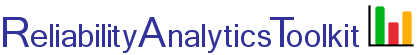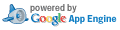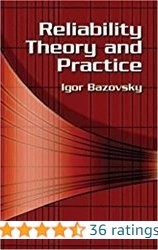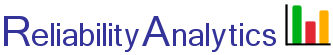# Sequential Reliability Testing

This tool provides the ability to plan a sequential reliability demonstration test for verification of equipment mean time between failure (MTBF), assuming an exponential failure distribution. Input parameters include the following:

• Lower test MTBF(θ1). The test plan will reject an item whose true MTBF is θ1 with a probability of 1 - β.
• Upper test MTBF(θ0). The test plan will accept an item whose true MTBF is θ0 with a probability of 1 - α.   θ0 = d * θ1.
• Discrimination ratio (d). The discrimination ratio is one of the standard test plan parameters which establishes the test plan envelope. d = θ01.
• Consumer’s risk (β). The consumer’s risk is the probability of accepting an equipment with a true MTBF equal to the lower test MTBF (θ1).
• Producer’s risk (α). The producer’s risk is the probability of rejecting an equipment with a true MTBF equal to the upper test MTBF (θ0).
• True MTBF. The MTBF that would be observed if an infinite number of units were tested for an infinite amount of time.
See reference 1, chapter 24, for a more detailed discussion on sequential reliability testing and this blog post for an example. The Operating Characteristic (OC) curve is generated using Equation 7 in Reference 6.

## Calculation Inputs:

1. Lower test MTBF (θ1, hours):
2. Nominal decision risks (α & β)
3. Discrimination ratio (d)

4. Chart overlay options:

4A. Comma separated value failure times, measured in cumulative unit-hours (accounting for time accumulated on all test units from test start).

4B. True MTBF (hours): Number of tests:

## Featured Reference:Reliability Theory and Practice

Toolkit Home